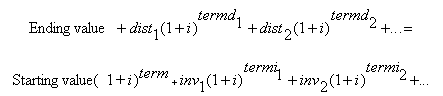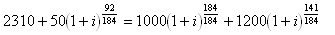Return On Investment (ROI) Yields

The yield that your invested monies have earned. This yield depends on the performance of the investment and when you made your purchases and redemptions. This yield is also sometimes referred to as the Internal Rate of Return (IRR) or Money-Weighted Return.  See Yield Calculations for a discussion of the differences between ROI and TWR yields.  ROI can be displayed for any investment, symbol, asset type, investment goal, sector, investment type, currency, or sub-portfolio.  The formula for ROI yield is:where the yield is 'i', and where each term is the number of years between the ending date of the yield term (the date the yield is being calculated 'to') and the date of the particular transaction. The variables inv1 and dist1 represent investments and distributions, respectively. A redemption is a negative investment, where a purchase is a positive investment. All investments and distributions from the beginning yield term date (inclusive) to the ending yield term date (inclusive) are included in the calculation. The Ending value is the closing value of the investment on the ending date of the yield term. The Starting value is the opening value of the investment on the beginning date of the yield term. (This opening value is the same as the closing value of the previous day.)  If either the Starting value or Ending value are negative, a yield cannot be calculated, and will be reported as "N/A".

Annualizing:

By default, yields for terms less than one year are not annualized, and yields for terms greater than one year are average annualized.  Whether or not to average annualize yields can be controlled from the Preferences - Yield dialog.

Accrued Interest:

By default, accrued interest is included in yield calculations.  Accrued interest as of the beginning of the yield term is added into the "Starting value" figure.  Accrued interest as of the end of the yield term is added into the "Ending value" figure.  Whether or not to include accrued interest in the yield calculations can be controlled from the Preferences - Yield dialog.

Example Calculation:
Assume we are calculating the 6 month (non-annualized) ROI yield ending on 12/31/2009.  The date range for this yield term would be 7/1/2009 through 12/31/2009, inclusive of gains and any transactions on both the starting and ending dates.  The closing value of this hypothetical investment on 6/30/2009 was \$1,000, which is the opening value on the beginning of yield term, 7/1/2009.  The closing value of the investment on 12/31/2009 was \$2,310.  The investment did not accrue interest.  There was one purchase within this time period for \$1,200 on 8/12/2009, and a distribution was received in cash on 9/30/2009 for \$50.  The equation to solve for this yield would look like:where there are 184 days from 7/1/2009 through 12/31/2009, there are 92 days from the time of the distribution to the ending yield date, and there are 141 days from the purchase to the ending yield date.

Fund Manager iteratively solves this equation for 'i', reporting a 6 month non-annualized ROI yield value of 8.48%.

Logging Details:

Fund Manager can optionally log the ROI yield equation to an external log file.  See General Preferences / Yields.